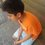# Trigonometric Identity

We know that $$\cos { 2x } =\cos ^{ 2 }{ x } -\sin ^{ 2 }{ x }$$

This is just a proof I have made. I don't know if it already exists but I just wanted to share it with you.

${ \left( { e }^{ ix } \right) }^{ 2 }={ e }^{ 2ix }\\ \\ { \left( { e }^{ ix } \right) }^{ 2 }={ e }^{ i\times 2x }\\ \\ { \left( \cos { x } +i\sin { x } \right) }^{ 2 }=cos2x+isin2x\\ \\ \cos ^{ 2 }{ x } +{ i }^{ 2 }\sin ^{ 2 }{ x } +2i\cos { x } \sin { x } =cos2x+isin2x\\ \\ \cos ^{ 2 }{ x } +\left( -1 \right) \sin ^{ 2 }{ x } +i\times 2\cos { x } \sin { x } =cos2x+isin2x\\ \\ \cos ^{ 2 }{ x } -\sin ^{ 2 }{ x } +i\sin { 2x } =cos2x+isin2x\\ \\ So,\quad \cos { 2x } =\cos ^{ 2 }{ x } -\sin ^{ 2 }{ x }$Note by Archit Boobna
6 years, 2 months ago

This discussion board is a place to discuss our Daily Challenges and the math and science related to those challenges. Explanations are more than just a solution — they should explain the steps and thinking strategies that you used to obtain the solution. Comments should further the discussion of math and science.

When posting on Brilliant:

• Use the emojis to react to an explanation, whether you're congratulating a job well done , or just really confused .
• Ask specific questions about the challenge or the steps in somebody's explanation. Well-posed questions can add a lot to the discussion, but posting "I don't understand!" doesn't help anyone.
• Try to contribute something new to the discussion, whether it is an extension, generalization or other idea related to the challenge.
• Stay on topic — we're all here to learn more about math and science, not to hear about your favorite get-rich-quick scheme or current world events.

MarkdownAppears as
*italics* or _italics_ italics
**bold** or __bold__ bold
- bulleted- list
• bulleted
• list
1. numbered2. list
1. numbered
2. list
Note: you must add a full line of space before and after lists for them to show up correctly
paragraph 1paragraph 2

paragraph 1

paragraph 2

[example link](https://brilliant.org)example link
> This is a quote
This is a quote
    # I indented these lines
# 4 spaces, and now they show
# up as a code block.

print "hello world"
# I indented these lines
# 4 spaces, and now they show
# up as a code block.

print "hello world"
MathAppears as
Remember to wrap math in $$ ... $$ or $ ... $ to ensure proper formatting.
2 \times 3 $2 \times 3$
2^{34} $2^{34}$
a_{i-1} $a_{i-1}$
\frac{2}{3} $\frac{2}{3}$
\sqrt{2} $\sqrt{2}$
\sum_{i=1}^3 $\sum_{i=1}^3$
\sin \theta $\sin \theta$
\boxed{123} $\boxed{123}$

## Comments

Sort by:

Top Newest

This also exists !!

- 6 years, 2 months ago

Log in to reply

But I thought it myself.

- 6 years, 2 months ago

Log in to reply

Ok ! Did you try all of my problems - new ones ?

- 6 years, 2 months ago

Log in to reply

Nice observational skill Archit :)

- 6 years, 2 months ago

Log in to reply

Thanks!

- 6 years, 2 months ago

Log in to reply

×

Problem Loading...

Note Loading...

Set Loading...